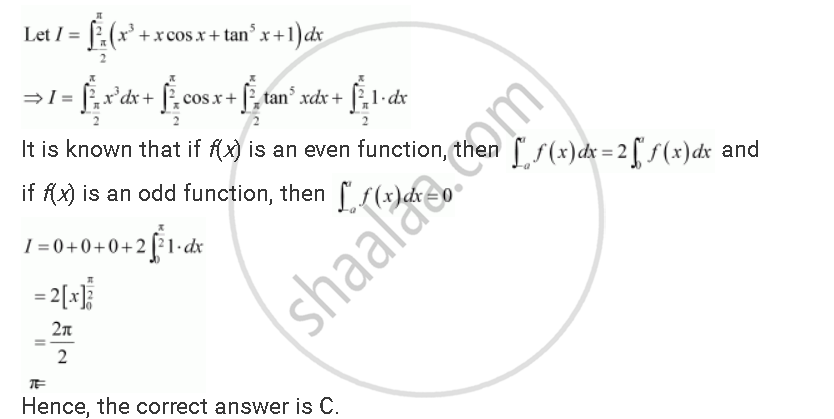Share

# Choose the Correct The Value of Int_(-pi/2)^(Pi/2) (X^3 + X Cos X + Tan^5 X + 1) Dx is - Mathematics

#### Question

Choose the correct The value of int_(-pi/2)^(pi/2) (x^3 + x cos x + tan^5 x + 1) dx  is

A. 0

B. 2

C. π

D. 1

#### SolutionIs there an error in this question or solution?

#### Video TutorialsVIEW ALL 

Solution Choose the Correct The Value of Int_(-pi/2)^(Pi/2) (X^3 + X Cos X + Tan^5 X + 1) Dx is Concept: Properties of Definite Integrals.
S